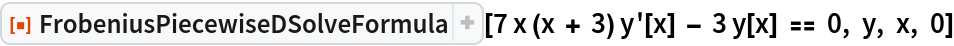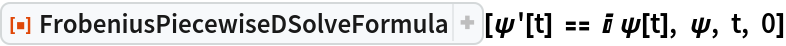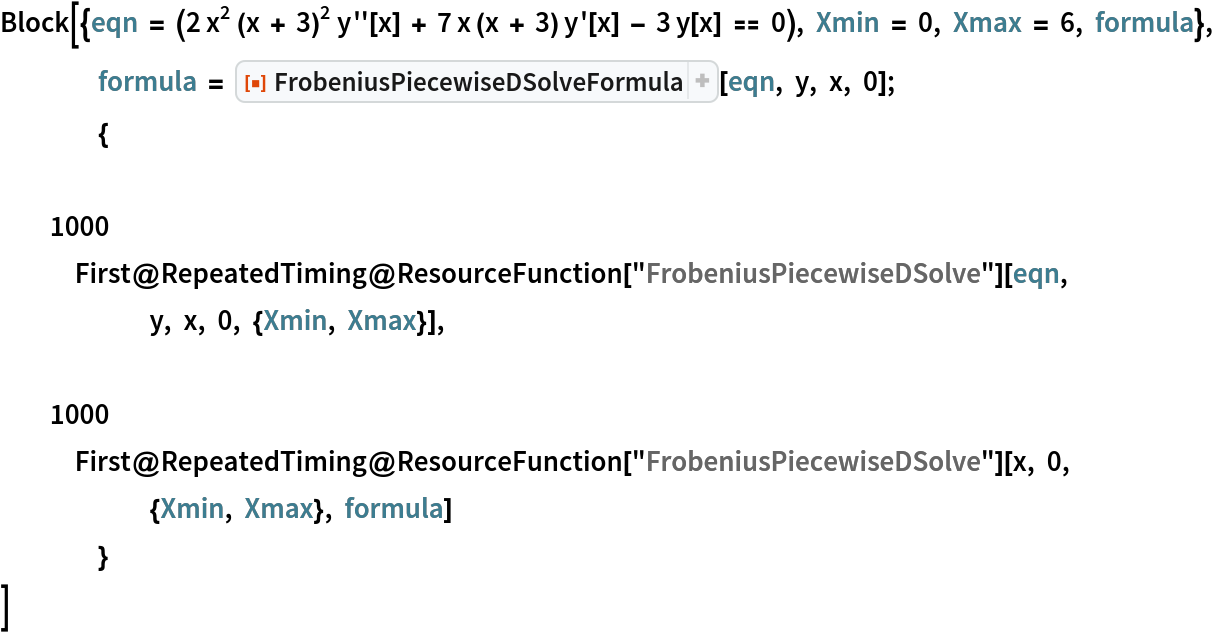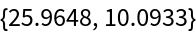Function Repository Resource:

# FrobeniusPiecewiseDSolveFormula

An auxiliary function for the resource function FrobeniusPiecewiseDSolve

Contributed by: Rauan Kaldybayev
 ResourceFunction["FrobeniusPiecewiseDSolveFormula"][eqn,u,x,x0] compute the formula for a piecewise Frobenius solution to a differential equation for the function u, with independent variable x.

## Details

The output is a formula to be fed into the resource function FrobeniusPiecewiseDSolve, which can in turn be used to solve ODEs.
The output of ResourceFunction["FrobeniusPiecewiseDSolveFormula"] doesn’t make much sense to a human and instead is meant to be fed into the resource function FrobeniusPiecewiseDSolve, which will in turn compute an actual solution.
When solving an ODE, the resource function FrobeniusPiecewiseDSolve first utilizes ResourceFunction["FrobeniusPiecewiseDSolveFormula"] to compute a formula for the solution. The formula is then used to calculate the approximate solutions. When a single ODE is to be solved many times with different parameters, computation time can be significantly reduced by computing the formula separately, using ResourceFunction["FrobeniusPiecewiseDSolveFormula"].
The function can solve linear homogeneous ODEs with polynomial coefficients.
Frobenius series solutions are quick to compute and very accurate. However, they generally only have a finite convergence radius, which limits their applications. This issue can be overcome with the introduction of the piecewise Frobenius method, which solves ODEs by "gluing" several series solutions together under the condition of continuity.
Read more about the working principle of the function in the related Wolfram Community post.

## Examples

### Basic Examples (1)

Compute the formula for a piecewise Frobenius solution to the ODE 7x(x+3)y'-3y=0 near the regular singular point x=0:

 In:=Out=### Scope (1)

The function works with complex numbers:

 In:=Out=### Properties and Relations (1)

The resource function FrobeniusPiecewiseDSolve first computes a formula and then uses it to calculate the solution. FrobeniusPiecewiseDSolveFormula allows us to compute the formula separately, which can help significantly reduce computation time when an ODE is to be solved repeatedly:

 In:=Out=## Publisher

Wolfram Summer Camp

## Version History

• 1.0.0 – 22 April 2021